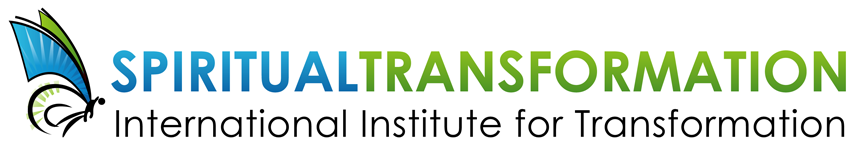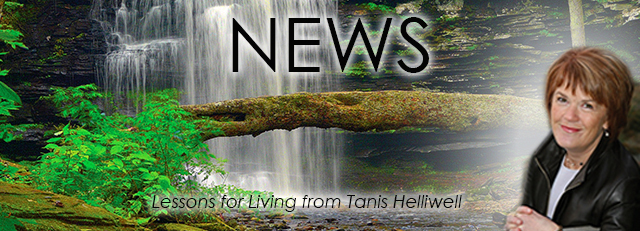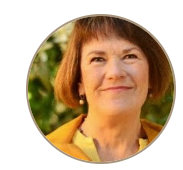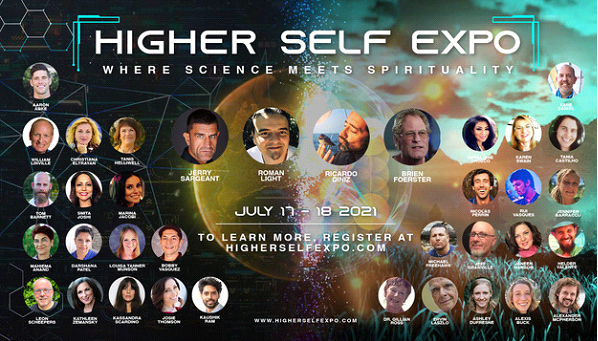# JULY 2021

 /* styles */ Dear Friend,
 /* styles */ This month will be a busy one! I will be speaking at a free 24-hour online conference on July 18th. Our IIT team is working hard on creating a new live online program launching this fall on Ancestor and Family Healing. Enjoy your summer sitting outside listening to my new audiobook!
 table div table+table+table+table+table div table{width:100%;padding:0}table div table+table+table+table+table div table img{width:96.23%;padding:0;float:none}table div table+table+table+table+table div table td{width:100%;padding:0 1.88% 18px}/* styles *//* styles */
 table div table+table+table+table+table+table+table div table{width:100%;padding:0}table div table+table+table+table+table+table+table div table img{width:96.23%;padding:0;float:none}table div table+table+table+table+table+table+table div table td{width:100%;padding:0 1.88% 18px}/* styles */# July 17 - 18, 2021

"Where Science Meets Spirituality"Tanis will be speaking on July 18thtable div table+table+table+table+table+table+table+table+table+table+table+table div table td,table.module-11{width:100%;padding:0}table div table+table+table+table+table+table+table+table+table+table+table+table div table{width:100%;float:none;margin-left:auto;margin-right:auto;padding:0}table div table+table+table+table+table+table+table+table+table+table+table+table div table a{border:0 none;text-decoration:none}table div table+table+table+table+table+table+table+table+table+table+table+table div table img{width:100%!important;border:0 none;text-decoration:none}/* styles */
 table div table+table+table+table+table+table+table+table+table+table+table+table+table div table{width:100%;padding:0}table div table+table+table+table+table+table+table+table+table+table+table+table+table div table img{width:96.23%;padding:0;float:none}table div table+table+table+table+table+table+table+table+table+table+table+table+table div table td{width:100%;padding:0 1.88% 18px}/* styles */# Begins September 2021

 table div table+table+table+table+table+table+table+table+table+table+table+table+table+table+table+table div table td,table.module-15{width:100%;padding:0}table div table+table+table+table+table+table+table+table+table+table+table+table+table+table+table+table div table{width:100%;float:none;margin-left:auto;margin-right:auto;padding:0}table div table+table+table+table+table+table+table+table+table+table+table+table+table+table+table+table div table a{border:0 none;text-decoration:none}table div table+table+table+table+table+table+table+table+table+table+table+table+table+table+table+table div table img{width:100%!important;border:0 none;text-decoration:none}/* styles */

# Are you or your family experiencing physical, emotional or mental difficulties stemming from past events or previous generations?

Our biological and spiritual ancestors are calling us to heal inherited patterns in our blood and spiritual lineage. Learn how our ancestors influence our lives today and how we can contact them for healing.

This new live online program will be offered in two separate parts. You may participate in one or both parts of the program - whatever will benefit you the most.

PART 1 will focus on our present, physical reality. We will study the gifts as well as the stuck, dark patterns of our family, tribe, and country ancestors and how these impact us today and ways to heal these imprinted cellular memories. Begins September 2021 for 9 weeks with a summary meeting bridge to Part 2.

PART 2 will focus on our ancestors from other realities such as our past lives, hybrid lineage, and spiritual ancestors. Begins January 2022 for 9 weeks with a summary meeting of healing the collective unconscious of humanity and our planet.

This program includes workbook modules, MP3/audio and video recordings for both parts of the program.

 /* styles */ (You will be the first to be notified of course schedules!)
 table div table+table+table+table+table+table+table+table+table+table+table+table+table+table+table+table+table+table+table+table div table{width:100%;padding:0}table div table+table+table+table+table+table+table+table+table+table+table+table+table+table+table+table+table+table+table+table div table img{width:96.23%;padding:0;float:none}table div table+table+table+table+table+table+table+table+table+table+table+table+table+table+table+table+table+table+table+table div table td{width:100%;padding:0 1.88% 18px}/* styles */# Summer with the Leprechauns is now an audio book

My bestselling classic is now available on Audible/Amazon and other fine audio book retailers.

 table div table+table+table+table+table+table+table+table+table+table+table+table+table+table+table+table+table+table+table+table+table+table+table div table td,table.module-22{width:100%;padding:0}table div table+table+table+table+table+table+table+table+table+table+table+table+table+table+table+table+table+table+table+table+table+table+table div table{width:100%;float:none;margin-left:auto;margin-right:auto;padding:0}table div table+table+table+table+table+table+table+table+table+table+table+table+table+table+table+table+table+table+table+table+table+table+table div table a{border:0 none;text-decoration:none}table div table+table+table+table+table+table+table+table+table+table+table+table+table+table+table+table+table+table+table+table+table+table+table div table img{width:100%!important;border:0 none;text-decoration:none}/* styles */
 /* styles */ Summer with the Leprechauns is an astonishing true story about one woman’s journey to Ireland where she lived in a cottage with leprechauns. These seldom-seen beings taught her about the evolution of elementals – the race to which leprechauns, faeries, elves, trolls belong. They explained the importance of humans and elementals working together for the betterment of both of their races and the Earth. Now available for download from Audible/Amazon for a new price of US \$19.95. Recording Length: 6 hrs and 19 mins Please consider leaving a review on Audible or wherever you get your audiobooks - many thanks!
 table div table+table+table+table+table+table+table+table+table+table+table+table+table+table+table+table+table+table+table+table+table+table+table+table+table+table+table+table div table{width:100%;padding:0}table div table+table+table+table+table+table+table+table+table+table+table+table+table+table+table+table+table+table+table+table+table+table+table+table+table+table+table+table div table img{width:96.23%;padding:0;float:none}table div table+table+table+table+table+table+table+table+table+table+table+table+table+table+table+table+table+table+table+table+table+table+table+table+table+table+table+table div table td{width:100%;padding:0 1.88% 18px}/* styles *//* styles */ Love as always,/* styles */ Tanis Helliwell info@iitransform.com International Institute for Transformation
 Like   Tweet   Pin   in